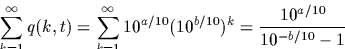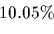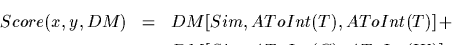Next: The FindBestPam Function Up: The Pairwise Comparison of Previous: The LogDelLocalRefine Function

# Estimating PAM Distances

The second type of computation we are interested in performing with alignments is the estimation of their PAM distance and variance. We require a little background before continuing. In the previous section, we discussed dynamic programming alignments where the score represents the logarithm of a probability (multiplied by 10). These computations were done for a single similarity matrix, hence for a single PAM distance. In other words, we are estimating the probability of two sequences having diverged from a common ancestor given a distance from this ancestor. It is natural to ask whether we can estimate this distance from the alignment itself. Since the scores are probabilities, we can immediately compute an estimate of the PAM distance by maximum likelihood. In other words, we select the PAM distance which gives an alignment with maximal score. As with all maximum likelihood estimators, this will be an unbiased estimator. Let Sp(a,b) be the score of aligning the sequences a and b at a PAM distance p. The maximum likelihood estimator, q is such thatThis estimation can be done by brute force or with Brent's minimization algorithm. In both cases we require several Dayhoff matrices (for various PAM distances). Since it is likely that we will compute more than one PAM distance in a normal session, it is more economical to pre-compute a dense set of similarity matrices for various PAM distances. The function CreateDayMatrices does exactly this, and assigns its result to the global variable DMS).

Finding the PAM distance which gives the maximum score requires between 13 and 15 alignments for arrays with up to 1000 entries.

Maximum likelihood however, does not allow us to compute the variance of the PAM distance. By assuming that the distribution of PAM distances for a random match is uniform between all possible values (for practical purposes, say up to PAM 1000), we can compute the PAM distance as an expected value:Recall that the score is 10 times the logarithm (base 10) of a probability. This observation allows us to compute, not only the first moment of p, the expected PAM distance, but all moments. In particularThe variance of the PAM distance can then be computed, and by using standard statistical tools we can estimate our confidence in E[p]. Letand the PAM distance is betweenand95% of the time.

The above integrals can be computed by standard methods of numerical integration. Due to the shape of this distribution, and its sharp decrease away from its maximum, the integration does not need to be done through the entire range, but only around its maximum value.Next: The FindBestPam Function Up: The Pairwise Comparison of Previous: The LogDelLocalRefine Function
Gaston Gonnet
1998-09-15